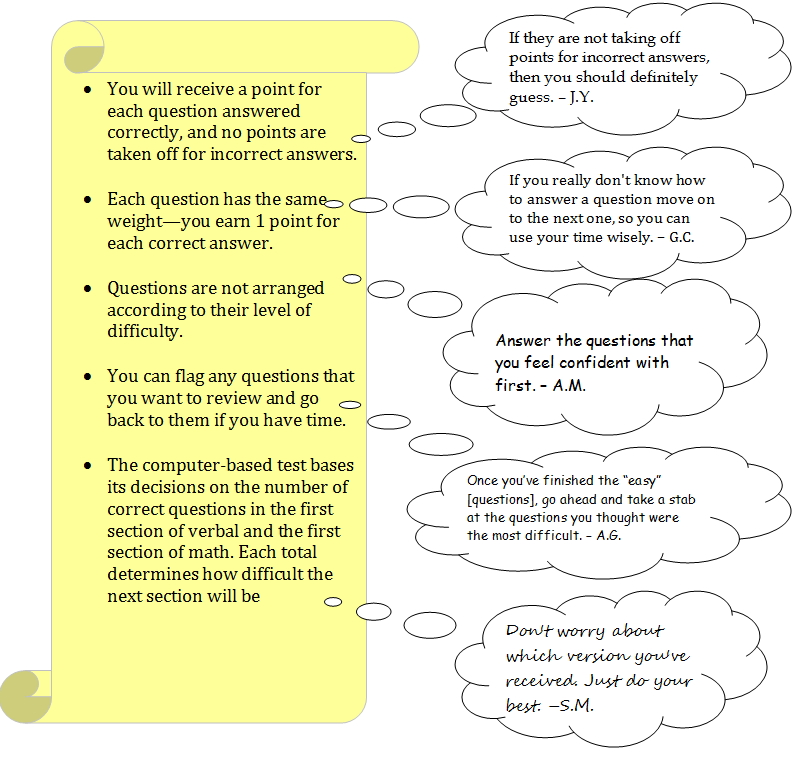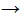05. Beat the Revised Scoring System

Beat the Revised Scoring System

You need to understand a system in order to figure out how to succeed within it. So, our staff writers reviewed the GRE's new scoring system and took note of any strategies that you can use to earn the most points as you work.More Details on the Computer-Based Test

• If the student did not answer enough questions correctly, he/she will receive a test with easier questions, but the student will not have the opportunity to earn a score as high as those who took the difficult section.
• If enough answers are correct, the computer will give the student a test with difficult questions. That student will then be able to maximize his/her score.

Our General Test-Taking Strategies In Review
(with an extra tip at the end as a bonus)

• Guess: Since ETS does not deduct points for incorrect answers, make sure to answer each question by the end of the exam -- even if you have to guess.
• Use Your Time Wisely: If you do not know the answer to a question, you can flag that question and move on to the next one.
• Go Easy: Focus on answering the easier problems first in order to maximize the number of points you’ll earn.
• Get Difficult: Once you have finished answering the easy questions, you can go back to work on the difficult questions.
• Back for Seconds: Do your best on each section, and do not worry about which version you have received. Both versions can lead to a solid score.
• A Note on Reviewing Answers: Most test prep sites suggest sticking with your first answer. That is important if you are stuck on a question, but you should go back and check it if you have time. For extra critical reading practice, please take a look at this article. It describes a study that questions the claim that we should always go with our first instincts:
http://www.apa.org/monitor/apr05/instincts.aspx

How Does Your Raw Score on Brainfuse Relate to the GRE's Score?

0 points = No Score (NS)

1 point = 130

40 points = 170 (the maximum score)

The Brainfuse Equivalent

To calculate your raw score on Brainfuse, you can follow these steps:

1. Let n = Your Brainfuse Score (posted on the top, left-hand corner of the Answer Summary Page)
2. Divide n by 12.
3. Round down to the nearest integer to find your raw score. (We'll let r = raw score)
4. Add r to 130 to see what your estimated scaled score would be.

Example:

Let's say that Sample Student has received a Brainfuse score of 370 as the total for both sections of quantitative reasoning.
1. Let n = 370
2. 370 divided by 12 = 30.83
3. Round that number down to the nearest whole number: 30
4. Raw Score = 30; let r = 30

130+ 30 = 160

Estimated Scaled Score = 160

You can then see the concordance information on the ETS's website to figure out how that score relates to the old 200 to 800 score on each section.  Right-click the following link to open it in a new window:

http://www.ets.org/gre/institutions/scores/interpretSample Student has received a 610 according to that chart.

Note that ETS will “equate” scores based on the second section's level of difficulty and a few other factors. So, these are just rough estimates that can help you set a goal.

The Analytical Writing Section

You will receive a score between "0" and "6" on the Analytical Writing section.